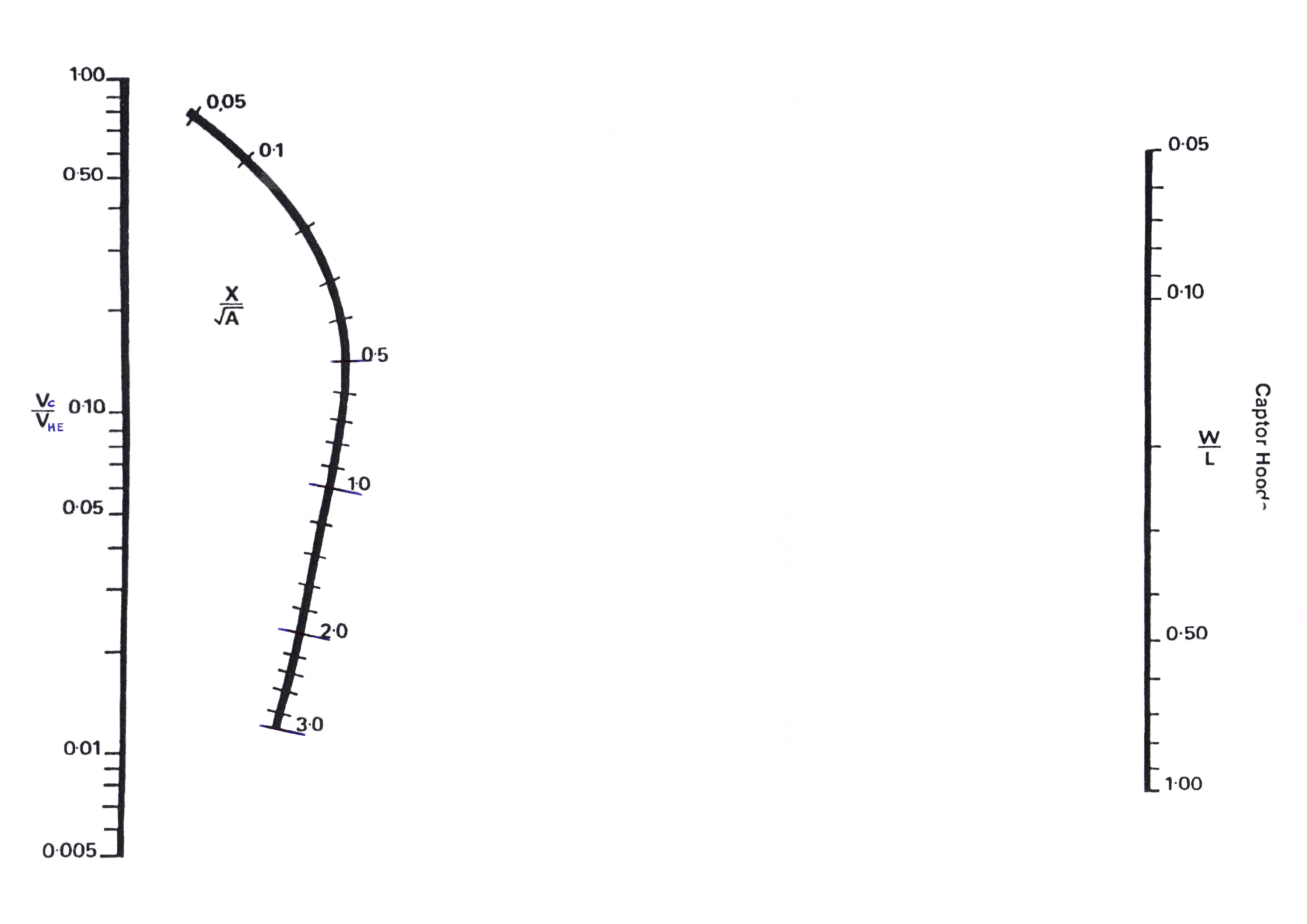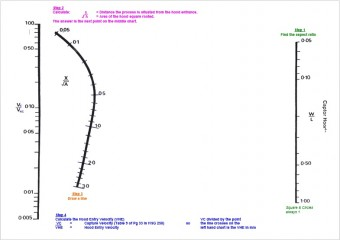## LEV Central

### The Knowledge Base for LEVFor Captor Hoods – Fletcher’s Nomogram chart for manual calculations of Hood Entry Velocity required to achieve a nominated minimum Captor Velocity at a chosen distance out from the hood (Captor Distance).

All units must be in metric

• Select hood dimensions and calculate Aspect Ration (small dimension divided by large)
• Mark Aspect Ration on right hand axis of chart
• Calculate hood area
• Take square root of hood area
• Decide Captor Distance required (X) on chart
• Divide Captor Distance (X) by the Square root of the hood area (A)
• Mark this figure on the middle line of the chart
• Draw a line with a ruler from the Aspect Ratio line through the middle line until it ‘hits’ the left hand vertical line
• This is the ration of the Captor Velocity divided by the Hood Entry (Face) velocity
• To get the Hood Entry velocity required divide your chosen Captor Velocity (from tables in HSG258) by the value you have just obtained from your drawn line where it intersected the vertical left hand chart lineAlternatively we have Fletchers Nomogram with notes (a how to guide):